RS Aggarwal Class 10 Solutions Chapter 4 Triangles

RS Aggarwal Class 10 Chapter 4 Triangles Solutions Free PDF

A triangle is simply a polygon which contains three edges and three vertices, making it one of the most quintessential shapes in geometry. Triangles are primarily classified on the basis of the length of the sides or by the internal angles that are formed. Euclid proposed the initial conceptualization of triangles and he assumes triangles to be two-dimensional plane figures. When combined, the sum of all the interior angles of a triangle in a Euclidean space is equal to 180 degrees.

Check out the RS Aggarwal Class 10 Solutions Chapter 4 Triangles below:

Question 1: D and E are points on the sides AB and AC respectively of a $\Delta$ABC such that DE || BC.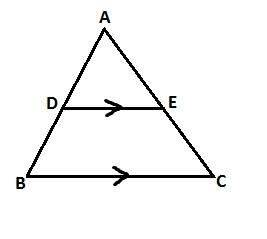(i) If AD = 3.6 cm, AB = 10 cm and AE = 4.5 cm, find EC and AC.

(ii) If AB = 13.3 cm, AC = 11.9 cm and EC = 5.1 cm, find AD.

(iii) If $\frac{AD}{DB}=\frac{4}{7}$ and AC = 6.6 cm, find AE.

(iv) If $\frac{AD}{AB}=\frac{8}{15}$ and EC = 3.5 cm, find AE.

Solution:

(i) In $\Delta$ABC, DE || BC, AD = 3.6 cm, AB = 10 cm, AE = 4.5 cm

Therefore, $\frac{AD}{DB}=\frac{AE}{EC}$ (By Thales theorem)

$\Rightarrow$ $\frac{3.6}{AB-AD}=\frac{4.5}{AC-AE}$

$\Rightarrow$ $\frac{3.6}{10-3.6}=\frac{4.5}{AC-4.5}$

$\Rightarrow$ $\frac{3.6}{6.4}=\frac{4.5}{AC-4.5}$

$\Rightarrow$ 3.6AC – 16.2 = 28.8

$\Rightarrow$ 3.6AC = 45

$\Rightarrow$ AC = 12.5 cm

Therefore, EC = AC – AE = 12.5 – 4.5 = 8 cm

Hence, AC = 12.5 cm and EC = 8 cm

(ii) In $\Delta$ABC, DE || BC, AB = 13.3 cm, AC = 11.9 cm and EC = 5.1 cm

Therefore, $\frac{AD}{DB}=\frac{AE}{EC}$ (By Thales theorem)

$\Rightarrow$ $\frac{AD}{AB-AD}=\frac{AC-EC}{EC}$

$\Rightarrow$ $\frac{AD}{13.5-AD}=\frac{11.9-5.1}{5.1}$

$\Rightarrow$ $\frac{AD}{13.5-AD}=\frac{6.8}{5.1}$

$\Rightarrow$ 5.1AD = 91.8 – 6.8AD

$\Rightarrow$ 11.9AD = 91.8

$\Rightarrow$ AD = $\frac{91.8}{11.9}$ = 7.7

(iii) In $\Delta$ABC, DE || BC, AC = 6.6 cm, $\frac{AD}{DB}=\frac{4}{7}$

Therefore, $\frac{AD}{DB}=\frac{AE}{EC}$ (By Thales theorem)

$\Rightarrow$ $\frac{4}{7}=\frac{AE}{AC-AE}$

$\Rightarrow$ $\frac{4}{7}=\frac{AE}{6.6-AE}$

$\Rightarrow$ 26.4 – 4AE = 7AE

$\Rightarrow$ 26.4 = 11AE

$\Rightarrow$ AE = $\frac{26.4}{11}$ = 2.4 cm

Hence, AE = 2.4 cm

(iv) In $\Delta$ABC, DE || BC, Given $\frac{AD}{AB}=\frac{8}{15}$, EC = 3.5 cm

Therefore, $\frac{AD}{DB}=\frac{AE}{EC}$ (By Thales theorem)

And AD = $\frac{8}{15}$AB [Given]

Therefore, $\frac{AD}{AB-AD}=\frac{AE}{3.5}$ [AD = $\frac{8}{15}$AB]

$\frac{\frac{8}{15}AB}{AB-\frac{8}{15}AB}=\frac{AE}{3.5}$

$\Rightarrow$ $\frac{\frac{8}{15}AB}{\frac{7}{15}AB}=\frac{AE}{3.5}$

$\Rightarrow$ $\frac{8}{7}=\frac{AE}{3.5}$

$\Rightarrow$ AE = $\frac{3.5\times 8}{7}$

$\Rightarrow$ AE = 4 cm

Hence, AE = 4 cm

Question 2: D and E are points on the sides AB and AC respectively of a $\Delta$ABC such that DE || BC.Find the value of x, when:

(i) AD = x cm, DB = (x -2) cm, AE = (x + 2) cm and EC = (x – 1) cm.

(ii) AD = 4 cm, DB = (x – 4) cm, AE = 8 cm and EC = (3x – 19) cm.

(iii) AD = (7x – 4) cm, AE = (5x – 2)cm, DB = (3x + 4) cm and EC = 3x cm.

Solution:

(i) D and E are points on the sides AB and AC respectively of a $\Delta$ABC such that DE || BC, AD = x cm, DB = (x – 2) cm, AE = (x + 2) cm, EC = (x – 1) cm

Therefore, $\frac{AD}{DB}=\frac{AE}{EC}$ (by thales theorem)

$\Rightarrow$ $\frac{x}{x-2}=\frac{x+2}{x-1}$

$\Rightarrow$ x(x – 1) = x2 – 4 $\Rightarrow$ x2 – x = x2 – 4

$\Rightarrow$ x = 4

(ii) In $\Delta$ABC, DE || BC, AD = 4 cm, DB = (x – 4) cm, AE = 8 cm, EC = (3x – 19) cm

$\frac{AD}{AB}=\frac{AE}{EC}$ (by thales theorem)

$\Rightarrow$ $\frac{4}{x-4}=\frac{8}{3x-19}$

$\Rightarrow$ 4(3x – 19) = 8(x – 4)

$\Rightarrow$ 12x – 76 = 8x – 32

$\Rightarrow$ 4x = 44

$\Rightarrow$ x = 11

$\frac{AD}{DB}=\frac{AE}{EC}$ (by thales theorem)

$\frac{7x-4}{3x+4}=\frac{5x-2}{3x}$

Hence, x = 11

(iii) In $\Delta$ABC, DE || BC, AD = (7x – 4) cm, AE = (5x – 2) cm, DB = (3x + 4) cm, EC = 3x cm

$\Rightarrow$ 21x2 – 12x = 15x2 – 6x + 20x – 8

$\Rightarrow$ 6x2 – 26x + 8 = 0

3x2 – 13x + 4 = 0

3x2 – 12x – x + 4 = 0

3x(x – 4) – 1(x – 4)

(x – 4)(3x – 1) = 0

x = 4 or x = $\frac{1}{3}$

If x = $\frac{1}{3}$, length of sides become negative

Hence, x = 4

Question 3: D and E are points on the sides AB and AC respectively of a $\Delta$ABC. In each of the following cases, determine whether DE || BC or not.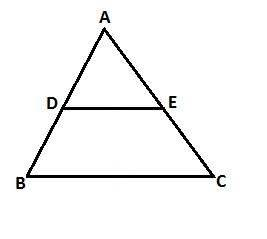(i) AD = 5.7 cm, DB = 9.5 cm, AE = 4.8 cm and EC = 8 cm.

(ii) AB = 11.7 cm, AC = 11.2 cm, BD = 6.5 cm and AE = 4.2 cm.

(iii) AB = 10.8 cm, AD = 6.3 cm, AC = 9.6 cm and EC = 4 cm.

(iv) AD = 7.2 cm, AE = 6.4 cm, AB = 12 cm and AC = 10 cm.

Solution:

Given: A $\Delta$ABC, in which D and E are points on the sides AB and AC respectively.

To prove: DE || BC

Proof:

(i) AD = 5.7 cm, DB = 9.5 cm, AE = 4.8 cm and EC = 8 cm

Since D and E are the points on AB and AC repectively.

Therefore, $\frac{AD}{DB}=\frac{AE}{EC}$

$\Rightarrow$ $\frac{5.7}{9.5}=\frac{4.8}{8}$

$\Rightarrow$ 0.6 = 0.6

Therefore, $\frac{AD}{DB}=\frac{AE}{EC}$ (each equal to 0.6)

Hence, by the converse of Thales theorem DE || BC

(ii) AB = 11.7 cm, AC = 11.2 cm, BD = 6.5 cm, AE = 4.2 cm

Since D and E are points on AB and AC respectively.

$\frac{AD}{DB}=\frac{AE}{EC}\Rightarrow \frac{AB-DB}{DB}=\frac{AE}{AC-AE}$

$\Rightarrow$ $\frac{11.7-6.5}{6.5}=\frac{4.2}{11.2-4.2}\Rightarrow \frac{5.2}{6.5}\neq \frac{4.2}{7}$

Hence, $\frac{AD}{DB}\neq \frac{AE}{EC}$

Hence, by the converse of Thales theorem DE is not parallel to BC.

(iii) AB = 10.8 cm, AD = 6.3 cm, AC = 9.6 cm, EC = 4 cm

Since D and E are the points on AB and AC respectively.

Therefore, $\frac{AD}{DB}=\frac{AE}{EC}$ [by thales theorem]

$\Rightarrow$ $\frac{AD}{AB-AD}=\frac{AC-EC}{EC}$

$\Rightarrow$ $\frac{6.3}{(10.8-6.3)}=\frac{(9.6-4.0)}{4}$

$\Rightarrow$ $\frac{6.3}{4.5}=\frac{5.6}{4}$

$\Rightarrow$ 1.4 = 1.4

Therefore, $\frac{AD}{DB}=\frac{AE}{EC}$ (each is equal to 1.4)

Hence by the converse of Thales theorem DE || BC

(iv) AD = 7.2 cm, AE = 6.4 cm, AB = 12 cm, AC = 10 cm

Since D and E are points on the side AB and AC respectively.

$\frac{AD}{DB}=\frac{AE}{EC}$ [by thales theorem]

$\frac{7.2}{AB-AD}=\frac{6.4}{AC-AE}$

$\Rightarrow$ $\frac{7.2}{12-7.2}=\frac{6.4}{10-6.4}$

$\Rightarrow$ $\frac{7.2}{4.8}=\frac{3.4}{3.6}$

but $\frac{3}{2}\neq \frac{16}{9}$

Hence, by the converse of Thales theorem DB is not parallel to BC

Question 4: In a $\Delta$ABC, AD is the bisector of $\angle$A.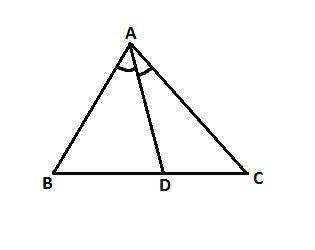(i) If AB = 6.4 cm, AC = 8 cm and BD = 5.6 cm, find DC.

(ii) If AB = 10 cm, AC = 14 cm and BC = 6 cm, find BD and DC.

(iii) If AB = 5.6 cm, BD = 3.2 cm and BC = 6 cm, find AC.

(iv) If AB = 5.6 cm, AC = 4 cm and DC = 3 cm, find BC.

Solution:

(i) AB = 6.4 cm, AC = 8 cm, BD = 5.6 cm

Let BC = x

Now, DC = (BC – BD) = (x – 5.6) cm

In $\Delta$ABC, AD is the base of $\angle$A

So, by the angle bisector theorem, we have

$\frac{BD}{DC}=\frac{AB}{AC}$

$\Rightarrow$ $\frac{5.6}{x-5.6}=\frac{6.4}{8}$

$\Rightarrow$ 6.4x – 35.84 = 44.8

$\Rightarrow$ 6.4x = 80.64

$\Rightarrow$ x = 12.6

Hence, BC = 12.6 cm and DC = (12.6 – 5.6) cm = 7 cm

(ii) AB = 10 cm, AC = 14 cm, BC = 6 cm

Let BD = x,

DC = (BC – BD) = (6 – x) cm

In $\Delta$ABC, AD is the bisector of $\angle$A

So, By angle bisector theorem,

We have, $\frac{BD}{DC}=\frac{AB}{AC}$

$\Rightarrow$ $\frac{x}{6-x}=\frac{10}{14}$

$\Rightarrow$ 14x = 60 – 10x

$\Rightarrow$ 24x = 60

$\Rightarrow$ x = 2.5

Hence, BD = 2.5 cm and DC = (6 – 2.5) cm = 3.5 cm

(iii) AB = 5.6 cm, BD = 3.2 cm and BC = 6 cm

DC = BC – BD = (6 – 3.2) cm = 2.8 cm

Let, AC = x,

In $\Delta$ABC, AD is the bisector of $\angle$A

So, by the angle bisector theorem we have

Therefore, $\frac{BD}{DC}=\frac{AB}{AC}$

$\Rightarrow$ $\frac{3.2}{2.8}=\frac{5.6}{x}$

$\Rightarrow$ x = $\frac{5.6\times 2.8}{3.2}=4.9$ cm

Hence, AC = 4.9 cm

(iv) AB = 5.6 cm, AC = 4 cm, DC = 3 cm

Let BD = x,

In $\Delta$ABC, AD is the bisector of $\angle$A

So, by the angle bisector theorem we have

Therefore, $\frac{BD}{DC}=\frac{AB}{AC}$

$\Rightarrow$ $\frac{x}{3}=\frac{5.6}{4}$

$\Rightarrow$ x = $\frac{5.6\times 3}{4}=4.2$ cm

Hence, BD = 4.2 cm

So BC = BD + AC = (4.2 + 3) cm

BC = 7.2 cm

Question 5: In the adjoining figure, ABCD is a trapezium in which CD || AB and its diagonals interact at O. If AO = (5x – 7) cm, OC = (2x + 1) cm, DO = (7x – 5) cm and OB = (7x + 1) cm, find the value of x.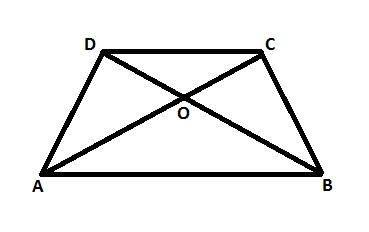Solution:

We know that CD || AB in trap ABCD and its diagonals interact at O.

Since the diagonals of a trapezium divide each other proportionally therefore, we have

$\frac{AO}{OC}=\frac{BO}{OD}$ (by thales theorem)

$\Rightarrow$ $\frac{5x-7}{2x+1}=\frac{7x+1}{7x-5}$

$\Rightarrow$ (5x – 7)()7x – 5) = (7x + 1)(2x + 1)

$\Rightarrow$ 35x2 – 25x – 49x + 35 = 14x2 + 7x + 2x + 1

$\Rightarrow$ 35x2 – 14x2 – 25x – 49x – 7x – 2x + 35 – 1 = 0

$\Rightarrow$ 21x2 – 83x + 34 = 0

Therefore, $x=\frac{83\pm \sqrt{(83)^{2}-4\times 21\times 34}}{21\times 2}$

= $\frac{83\pm \sqrt{4033}}{42}$

= $\frac{83\pm 63.51}{42}$

= $\frac{146.51}{42}$ or $\frac{19.49}{42}$ = 3.49 or 0.46

$\Rightarrow$ x = 0.46 or 3.49,

But x $\neq$ 0.46 as all sides should be positive.

Therefore, x = 3.49 cm

Question 6: $\Delta$ABC and $\Delta$DBC lie on the same side of BC, as shown in the figure. From a point P on BC, PQ || AB and PR || BD is drawn, meeting AC at Q and CD at R respectively. Prove that QR || AD.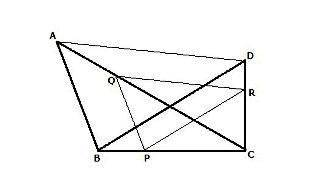Solution:

Given: $\Delta$ABC and $\Delta$DBC lie on the same side of BC. P is a point on BC, PQ || AB and PR || BD are drawn meeting AC at Q and CD at R respectively.

Proof: In $\Delta$ABC

PQ || AB

$\Rightarrow$ $\frac{CP}{PB}=\frac{CQ}{QA}$ ——– (1)(by thales theorem)

In $\Delta$BCD, PR || BD

Therefore, $\frac{CP}{PB}=\frac{CR}{RD}$ ——– (2)(by thales theorem)

From (1) & (2), we get:

$\frac{CQ}{QA}=\frac{CR}{RD}$

Hence, in $\Delta$ACD, Q and R the points in AC and CD such that

Therefore, $\frac{CQ}{QA}=\frac{CR}{RD}$

QR || AD (by the converse of Thales theorem)

Hence proved

Question 7: In the given figure, side BC of $\Delta$ABC is bisected at D and O is any point in AD. BO and CO produced meet AC and AB at E and F respectively, and AD is produced to X so that D is the mid-point of OX. Prove that AO: AX = AF: AB and show that EF || BC.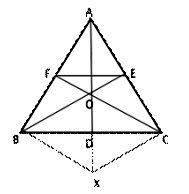Solution:

Given BD = CD and OD = DX

Join BX and CX

Thus, the diagonals of quad OBXC bisect each other

OBXC is a parallelogram

BX || CF and so, OF || BX

Similarly, CX || OE

In $\Delta$ABX, OF || BX

Therefore, $\frac{AO}{AX}=\frac{AF}{AB}$ —– (1)

In $\Delta$ACX, OE || XC

Therefore, $\frac{AO}{AX}=\frac{AE}{AC}$ —– (2)

From (1) & (2) we get:

$\frac{AF}{AB}=\frac{AE}{AC}$

Hence, EF || BC

Question 8: ABCD is a parallelogram in which P is the midpoint of DC and Q is a point on AC such that CQ = $\frac{1}{4}$AC. If PQ produced meets BC at R, prove that R is the mid-point of BC.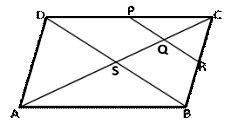Solution:

Given: ABCD is a parallelogram in which P is the mid-point of DC and Q is a point on AC such that CQ = $\frac{1}{4}$AC. PQ produced meets BC at R.

To prove: R is the mid-point of BC

Construction: Join BD

Proof: Since the diagonals of a parallelogram bisect each other at S such that

CS = ½ AC

Now, CS = ½ AC and CQ = $\frac{1}{4}$AC $\Rightarrow$ CQ = ½ CS

Therefore, Q is the mid-point of CS

So, PQ || DS

Therefore, QR || SB

In $\Delta$CSB, Q is the mid-point of CS and QR || SB.

So R is the midpoint of BC.

Question 9: In the adjoining figure, ABC is a triangle in which AB = AC. If D and E are points on AB and AC respectively such that AD = AE, show that the points B, C, E, and D are concyclic.Solution:

Given: ABC is a triangle in which AB = AC. D and E are points on AB and AC respectively such that AD = AE

To prove: AB = AC (given)

$\Rightarrow$ (AB – AD) = (AC – AE)

$\Rightarrow$ DE = EC

$\Rightarrow$ $\frac{AD}{AE}=\frac{DB}{EC}$ (each equal to 1)

$\Rightarrow$ DE || BC (by the converse of Thales’s theorem)

$\Rightarrow$ $\angle$DEC + $\angle$ECB = 180o

$\Rightarrow$ $\angle$DEC + $\angle$CBD = 180o [AB = AC $\Rightarrow$ $\angle$C = $\angle$BB]